## 3.7.2 LANGRETH Theorem

The next step is replacing contour by real time integrals in the DYSON equation. In that equation one encounters the following contour integrals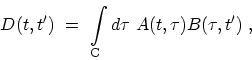(3.53)

and their generalizations involving products of three or more terms. To evaluate (3.53) one can assume that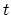is on the first half of the contour and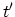is on the latter half. In view of the discussion of (3.51), we are thus analyzing a lesser function. The next step is to deform the contour as indicated in Fig. 3.10. Thus (3.53) becomes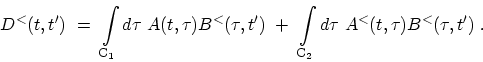(3.54)

Here, in appending the label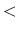to the function B in the first term we made use of the fact that as long as the integration variable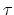is confined on the contour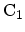it is less than(in the contour sense) . A similar argument applies to the second term. Considering the first term in (3.54) the integration can be split into two parts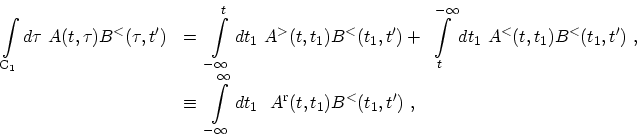(3.55)

where the definition of the retarded function (3.52) has been used. A similar analysis can be applied to the second term involving contour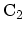, where the advanced function is generated. Putting the two terms together, one gets the first of LANGRETH's results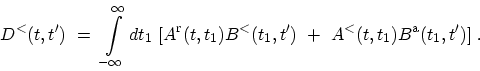(3.56)

The same result applies for the greater function just by replacing thelabels by the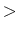labels. It is easy to generalize the result (3.56) to a product of three functions. The retarded and analogously the advanced component of a product of functions defined on the contour can be derived by repeated use of the definitions (3.51) and (3.52) and the result (3.56).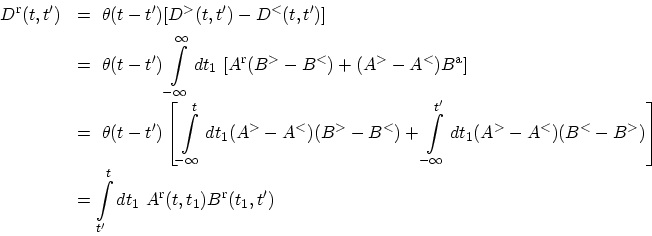(3.57)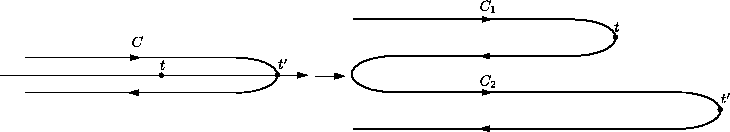In the self-energies another structure occurs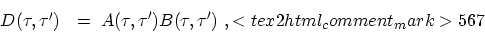(3.58)

whereand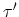are contour variables. The derivation of the required formula is similar to the analysis presented above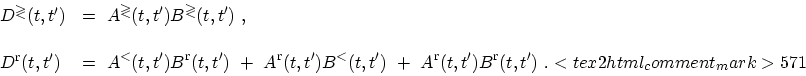(3.59)

The rules provided by the LANGRETH theorem are summarized in Table 3.1.

Table 3.1: Rules for analytic continuation derived from the LANGRETH theorem.
 Contour Real axis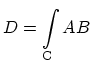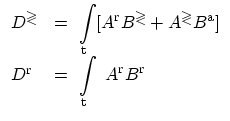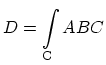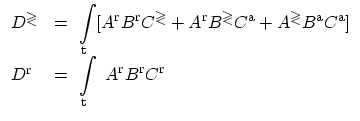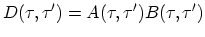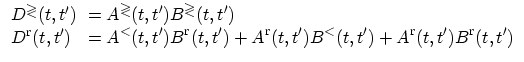M. Pourfath: Numerical Study of Quantum Transport in Carbon Nanotube-Based Transistors